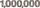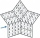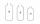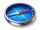# Pythagoras contest + Mathematical Olympiad - math problems

#### Number of problems found: 8

• One millionWrite the million number (1000000) using only nine numbers and algebraic operations plus, minus, times, divided, powers, and squares. Find at least three different solutions.
• All pairsDetermine all pairs (m, n) of natural numbers for which is true: m s (n) = n s (m) = 70, where s (a) denotes the digit sum of the natural number a.
• Alarm clockThe old watchmaker has a unique digital alarm in its collection that rings whenever the sum of digits of the alarm is equal to 21. Find out when the alarm clock will ring. What is their number? List all options . ..
• BicyclesYou're the owner of the transport 's learning playground. Buy bicycles of two colors but you've got to spend accurately 120000 Kč. Blue bike costs 3600Kč and red bicycle 3200Kč.
• FluidWe have vessels containing 7 liters, 5 liters and 2 liters. Largest container is filled with fluid the others empty. Can you only by pouring get 5 liters and two 1 liter of fluid? How many pouring is needed?
• Skiing meetingOn the skiing meeting came four friends from 4 world directions and led the next interview. Charles: "I did not come from the north or from the south." Mojmir "But I came from the south." Joseph: "I came from the north." Zdeno: "I come from the south." We
• Equilateral triangle ABCIn the equilateral triangle ABC, K is the center of the AB side, the L point lies on one-third of the BC side near the point C, and the point M lies in the one-third of the side of the AC side closer to the point A. Find what part of the ABC triangle cont
• Self-counting machineThe self-counting machine works exactly like a calculator. The innkeeper wanted to add several three-digit natural numbers on his own. On the first attempt, he got the result in 2224. To check, he added these numbers again and he got 2198. Therefore, he a

We apologize, but in this category are not a lot of examples.
Do you have an interesting mathematical word problem that you can't solve it? Submit a math problem, and we can try to solve it.

We will send a solution to your e-mail address. Solved examples are also published here. Please enter the e-mail correctly and check whether you don't have a full mailbox.

Please do not submit problems from current active competitions such as Mathematical Olympiad, correspondence seminars etc...

Pythagoras contest - math problems. Mathematical Olympiad - math problems.